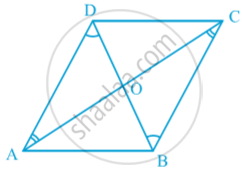Share

# Properties of a Parallelogram - The Diagonals of a Parallelogram Bisect Each Other.

Course

#### theorem

Theorem : The diagonals of a parallelogram bisect each other.
proof :Since ABCD is a parallelogram
therefore  AB || DC and AD || BC
Now, AB parallel to DC and AC is the transversal intersecting them at A and C respectively.
∠BAC =∠ DCA (Alternate interior angles)
Thus, ∠ BAO = ∠ DCO ... (1)
As , AB || DC and BD intersect them at B and D respectively.
∠ ABD= ∠CDB (Alternate interior angles)
So, ∠ ABD= ∠CDO   ...(2)
Now , In triangle AOB and Triangle COD , we have
∠BAO = ∠  DCO [From (1)]
AB = CD ( Opposites sides of a parallelogram )
∠ ABO =∠ CDO  [From (2)]
therefore  ∆ AOB ≅ ∆ COD   (by ASA congruence criterion)
Thus , OA = OC and OB = OD (C.P.C.T)

### Shaalaa.com

Theorem : The diagonals of a parallelogram bisect each other. [00:14:59]
S
0%

S